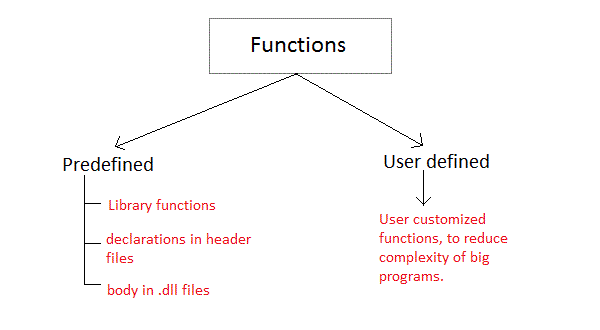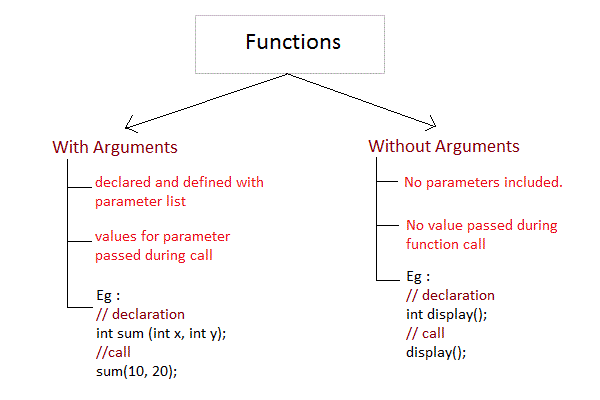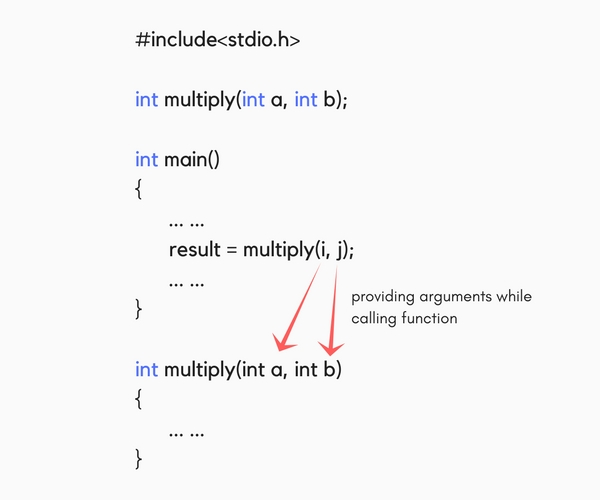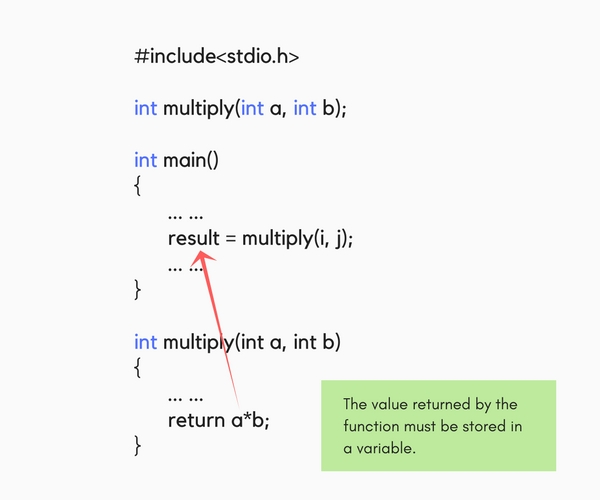Figure out what the program does and the output

Again figure out what the program does and the output

Compare above two program , you will find the use of function .

# # C Function Give name to the task

A function is a `block of code` that performs specific task. Tasks of a complex program are divided into functions and these functions are called wherever needed in the program. Dividing complex problem into small components makes program `easy` to `understand`and `use`.

There are two types of functions in C programming:

1. Standard Library functions
2. User defined functionsQ) List the Standard Library functions that you have used until ?

## # Standard Library Functions

The standard library functions are `built-in functions` in C programming to handle tasks such as mathematical computations, I/O processing, string handling etc.

## # User defined Functions

The functions created by a user are called user-defined functions.

Any user-defined function has `3` parts:

1. Function Declaration
2. Function Definition
3. Function call

### # Function Declaration

General syntax for function declaration is,

``````returntype functionName(type1 parameter1, type2 parameter2,...);
``````
1

Like any `variable` or an `array`, a function must also be declared before its used. Function declaration informs the compiler about the function name, parameters is accept, and its return type. The actual body of the function can be defined separately. Function declaration consists of 4 parts.

1. returntype(`eg: void , int , char`)

When a function is declared to perform some sort of calculation or any operation and is expected to provide with some result at the end, in such cases, a return statement is added at the end of function body. Return type specifies the type of value(`int`, `float`, `char`, `double`) that function is expected to return to the program which called the function.

Note: In case your function doesn't return any value, the return type would be `void`.

1. function name (`same like variable name`)
2. parameter list (`variable declaration`)
3. terminating semicolon(`;`)

### # Function Definition

In function definition, we define `name` and `tasks` performed by the function. This is where we define what the function does.

``````void functionName()
{
function statements;
}
``````
1
2
3
4

The body is enclosed within curly braces `{ ... }` and consists of three parts.

1. local variable declaration(if required).
2. function statements to perform the task inside the function.
3. a return statement to return the result evaluated by the function(if return type is `void`, then no return statement is required).

### # Function Call

Function call is simply `calling` the function in main program to perform its task.

``````functionName();
``````
1

When a function is called, control of the program gets transferred to the function.

A basic example for user-defined function is given below:

Tasks

1. Find three part of user defined function.

## # Passing Arguments to a function

Arguments are the values specified during the function call, for which the formal parameters are declared while defining the function.It is possible to have a function with parameters but no return type. It is not necessary, that if a function accepts parameter(s), it must return a result too.While declaring the function, we have declared two parameters a and b of type int. Therefore, while calling that function, we need to pass two arguments, else we will get compilation error. And the two arguments passed should be received in the function definition, which means that the function header in the function definition should have the two parameters to hold the argument values. These received arguments are also known as formal parameters. The name of the variables while declaring, calling and defining a function can be different.

## # Returning a value from function

A function may or may not `return` a result. But if it does, we must use the `return` statement to output the result. return statement also ends the function execution, hence it must be the last statement of any function. If you write any statement after the `return` statement, it won't be executed.The data type of the value returned using the return statement should be same as the `return` type mentioned at function declaration and definition. If any of it mismatches, you will get compilation error.

In the next tutorial, we will learn about the different types of user defined functions in C language and the concept of Nesting of functions which is used in recursion.

## # Advantages of user-defined functions

1. The program will be easier to `understand`, `maintain` and `debug`.
2. `Reusable codes` that can be used in other programs
3. A large program can be divided into smaller `modules`. Hence, a large project can be divided among many `programmers`.